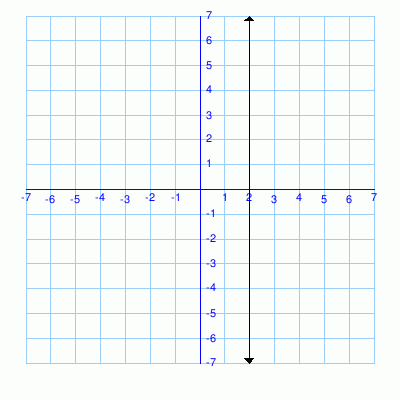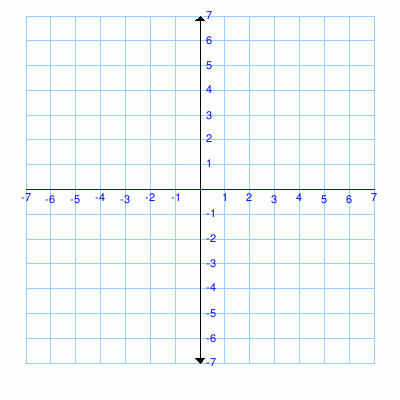MathScore EduFighter is one of the best math games on the Internet today. You can start playing for free!

## Domain and Range - Sample Math Practice Problems

The math problems below can be generated by MathScore.com, a math practice program for schools and individual families. References to complexity and mode refer to the overall difficulty of the problems as they appear in the main program. In the main program, all problems are automatically graded and the difficulty adapts dynamically based on performance. Answers to these sample questions appear at the bottom of the page. This page does not grade your responses.

See some of our other supported math practice problems.

### Complexity=1, Mode=ordpair

Find the domain and range. Give answers in ascending order. Example: {-2, 1, 5} but not {1, -2, 5}.

 1 { (0, 15), (4, 6), (4, 7), (3, 3) } Domain: Range: 2 { (3, 6), (10, -5), (5, -2), (7, 1) } Domain: Range:

### Complexity=1, Mode=graph

Find the domain and range.
Use curly braces like {-2, 1, 5} for specific numbers, and parentheses like (-∞, ∞) for spans whose ends are exclusive.
Type "inf" for ∞, like (-inf, inf) for (-∞, ∞).

 1Domain: Range: 2Domain: Range:

### Complexity=1, Mode=exp

Find the domain and range.
Use curly braces like {-2, 1, 5} for specific numbers, and parentheses like (-∞, ∞) for spans whose ends are exclusive.Use square brackets like [0, 5] for spans whose ends are inclusive.
Type "inf" for ∞, like (-inf, inf) for (-∞, ∞). For the union symbol, use capital "U" or lowercase "u".

 1 f(x) = √5x - 15 Domain: Range: 2 f(x) = √2x - 18 Domain: Range:

### Complexity=1, Mode=ordpair

Find the domain and range. Give answers in ascending order. Example: {-2, 1, 5} but not {1, -2, 5}.

1{ (0, 15), (4, 6), (4, 7), (3, 3) } Domain: Range:
2{ (3, 6), (10, -5), (5, -2), (7, 1) } Domain: Range:

### Complexity=1, Mode=graph

Find the domain and range.
Use curly braces like {-2, 1, 5} for specific numbers, and parentheses like (-∞, ∞) for spans whose ends are exclusive.
Type "inf" for ∞, like (-inf, inf) for (-∞, ∞).

1Domain: Range:
2Domain: Range:

### Complexity=1, Mode=exp

Find the domain and range.
Use curly braces like {-2, 1, 5} for specific numbers, and parentheses like (-∞, ∞) for spans whose ends are exclusive.Use square brackets like [0, 5] for spans whose ends are inclusive.
Type "inf" for ∞, like (-inf, inf) for (-∞, ∞). For the union symbol, use capital "U" or lowercase "u".

1f(x) = √5x - 15 Domain: Range:
Solution
Domain is all possible x's. For square roots, the expression under the radical must be greater or equal to zero.
Set the expression greater or equal to 0 and solve:
 5x - 15 ≥ 0 5x ≥ 15 x ≥ 3
The domain contains all values of x where x ≥ 3.

Range is all possible y's. Square roots are greater or equal to zero.
So the range contains all values of f(x) where f(x) ≥ 0.

2f(x) = √2x - 18 Domain: Range:
Solution
Domain is all possible x's. For square roots, the expression under the radical must be greater or equal to zero.
Set the expression greater or equal to 0 and solve:
 2x - 18 ≥ 0 2x ≥ 18 x ≥ 9
The domain contains all values of x where x ≥ 9.

Range is all possible y's. Square roots are greater or equal to zero.
So the range contains all values of f(x) where f(x) ≥ 0.